# Basic Arithmetic : Multiplication with Whole Numbers

## Example Questions

### Example Question #12 : Operations With Whole Numbers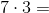What is the solution of the above equation?Explanation: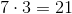We can see this by implementing objects.groups ofobjects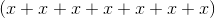^ We can see that there are 21 objects.

### Example Question #12 : Whole Numbers

What is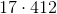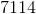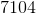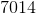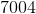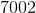Explanation: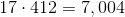.

Use a calculator to check your work if allowed, but if not, then I'd reccommend the following method, breaking the problem down into smaller pieces.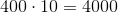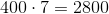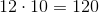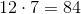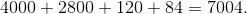### Example Question #1 : Multiplication With Whole Numbers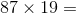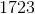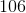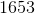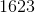Explanation: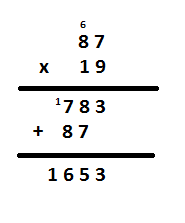Start with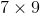. That gives. Write the ones' place down underneath theand theand carry theto the next place.

Next, do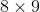. That gives. However, remember to add theyou carried over from the previous step.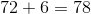Now, write down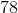down next to the. Make sure theis lined up beneath theandand theis in the hundreds' place.

Now, do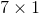. Because we are multiplying using the tens' place of, you need to make sure that you write down the product ofandin the tens' place, underneath thefrom your previous answer.

Next, do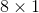. Write this product () in the hundreds' place.

Finally, add these two numbers together.

### Example Question #1 : Multiplication With Whole Numbers

What is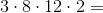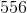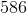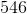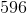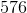Explanation:

You can simplify this problem: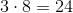, and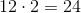, so if you simplify those parts of the equation, you are left with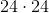.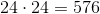### Example Question #4 : Multiplication With Whole Numbers

Solve for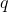: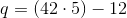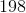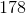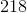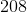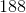Explanation:

To solve this equation, follow order of operations:

First we do the calculations inside the parentheses.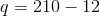Then we subtract to get our final answer.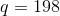### Example Question #22 : Whole Numbers

Solve for: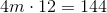Explanation:

To solve for m we need to isolate m on one side of the equation and all other numbers on the other side of the equation.Our first step is to divide by 12 on both sides. Remember to undo an operation from one side of the equation and cancel out a number we need to perform the opposite operation. If you have a number multiplied by another number or a variable you need to divide to cancel out that said number.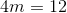Then we divide by 3 to get our final answer.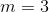### All Basic Arithmetic Resources